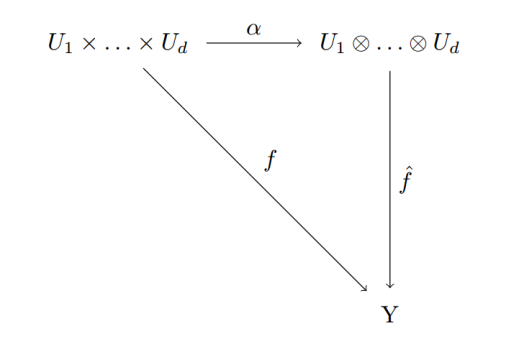Category Theory

# Categories – Exercise 1[Two-out-of-three property]  Let f: A→B and g: B→ C be two morphisms. If $$g$$ and $$gf$$ are isomorphisms, then so is f$$. Solution: There exists h: C→A such that$$(gf)\circ h = I_{C}$$. By hypothesis,$$g$$is an isomorphism, so $(gf)\circ h = I_{C}\Longrightarrow$ $g^{-1}(gf)\circ h = g^{-1}I_{C}=g^{-1} \Longrightarrow$ $f\circ h \circ g= g^{-1}g=I_{C}\Longrightarrow$ $f\circ h=I_{C}.$ We have the same script for the left inverse: $h\circ (gf) = I_{A}$ From the uniqueness of$$f^{-1}$$,$$f^{-1}=(h\circ g).

Tags: